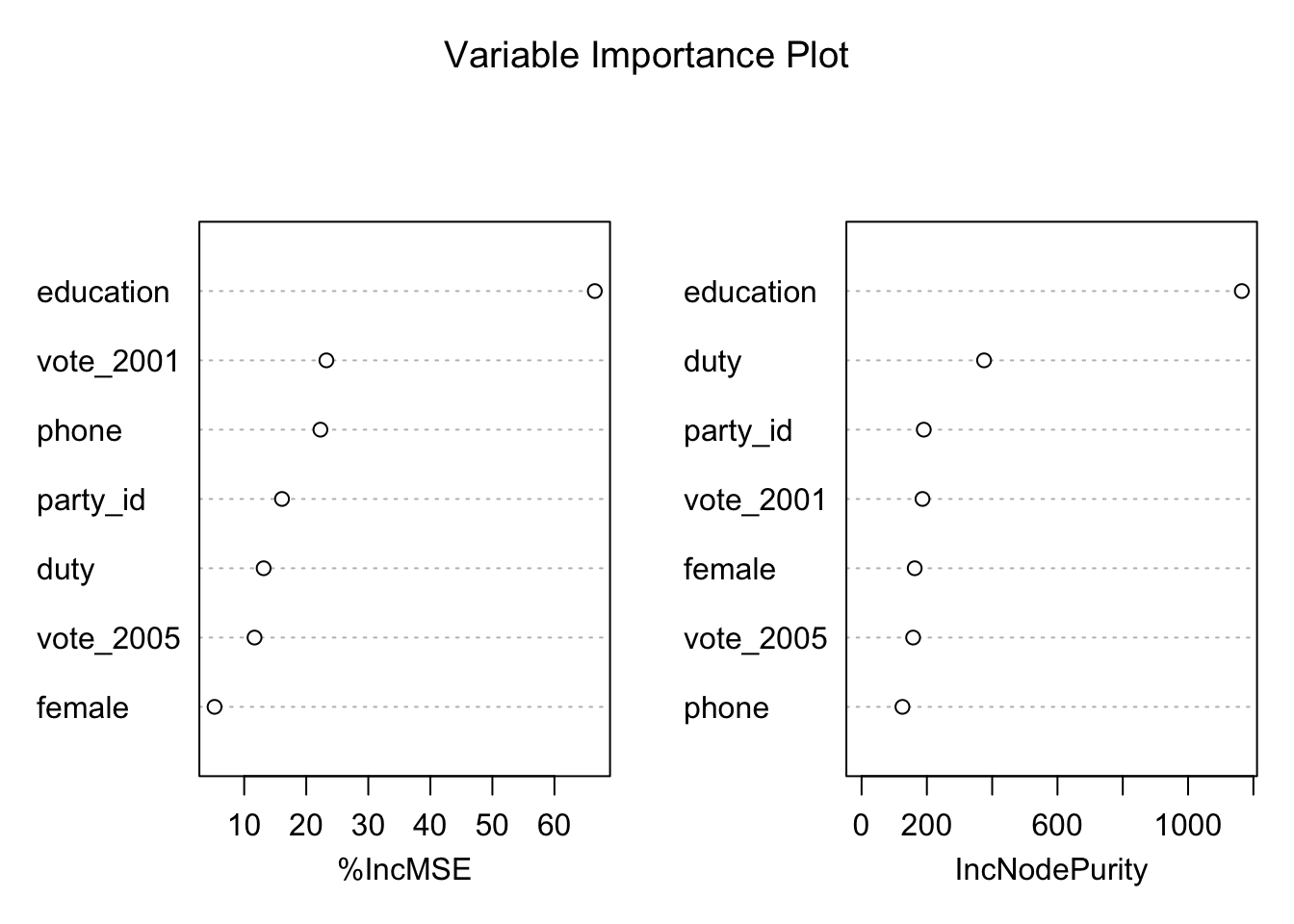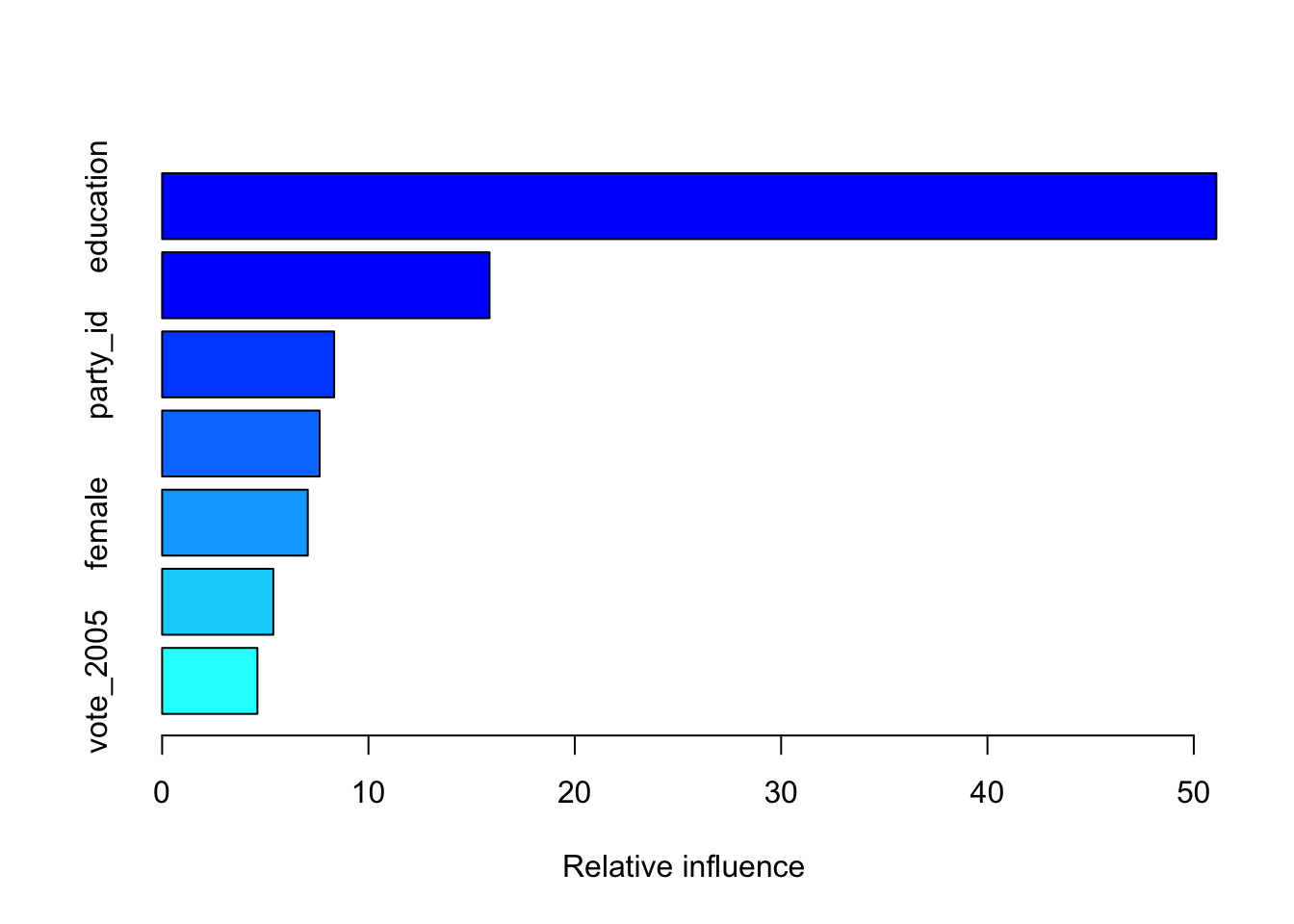## Bagging

Here we apply bagging to the 2005 BES survey data, using the randomForest package in R. Recall that bagging is a special case of a random forest with $$m = p$$. Therefore, the randomForest() function can be used to perform both random forests and bagging. We perform bagging as follows:

library(randomForest)

# Load data set
load("bes_2005.RData")

# Create training and test sets
set.seed(1234)
train <- sample(1:nrow(bes_2005), size = as.integer(nrow(bes_2005) / 2))
bes_2005_test <- bes_2005[-train, ]
vote_2005_test <- bes_2005$vote_2005[-train] # Bagging set.seed(1234) bag_1 <- randomForest(household_income ~ ., data = bes_2005, subset = train, mtry = 7, importance = TRUE) bag_1 ## ## Call: ## randomForest(formula = household_income ~ ., data = bes_2005, mtry = 7, importance = TRUE, subset = train) ## Type of random forest: regression ## Number of trees: 500 ## No. of variables tried at each split: 7 ## ## Mean of squared residuals: 8.813623 ## % Var explained: 14.23 Note that the argument mtry = 7 indicates that all 7 predictors should be considered for each split of the tree—in other words, that bagging should be done. How well does this bagged model perform on the test set? # Predict outcomes bag_1_pred <- predict(bag_1, newdata = bes_2005_test) # MSE household_income_test <- bes_2005$household_income[-train]
mean((bag_1_pred - household_income_test)^2)
##  11.3849

## Random Forest

Growing a random forest proceeds in exactly the same way, except that we use a smaller value of the mtry argument. By default, randomForest() uses $$p/3$$ variables when building a random forest of regression trees, and $$\sqrt{p}$$ variables when building a random forest of classification trees. Here we use mtry = 3.

# Random forest
set.seed(1234)
rf_1 <- randomForest(household_income ~ ., data = bes_2005, subset = train,
mtry = 3, importance = TRUE)

# Predict outcomes
rf_1_pred <- predict(rf_1, newdata = bes_2005_test)

# MSE
mean((rf_1_pred - household_income_test)^2)
##  10.49424

The test set MSE is 10.49. This indicates that random forests yielded an improvement over bagging in this case. Using the importance() and varImpPlot() functions, we can view the importance of each variable.

# Variable importance
importance(rf_1)
##             %IncMSE IncNodePurity
## vote_2005 11.665744      157.8210
## vote_2001 23.246025      186.5598
## female     5.225216      162.7271
## party_id  16.091845      190.4920
## phone     22.284473      125.1718
## education 66.508981     1164.5620
## duty      13.134319      375.1277
# Variable importance plot
varImpPlot(rf_1, main = "Variable Importance Plot")## Boosting

We use the gbm() function from the gbm package to fit boosted regression trees to the 2005 BES survey data. We run gbm() with the option distribution = "gaussian" since this is a regression problem. If it were a binary classification problem, we would use distribution = "bernoulli". The argument n.trees = 5000 indicates that we want 5000 trees, and the option interaction.depth = 4 limits the depth of each tree. Note that the learning rate is related to the tree size: when the learning rate is smaller, we need more trees. The summary() function produces a relative influence plot and also outputs the relative influence statistics.

library(gbm)

# Boosting
set.seed(1234)

boost_1 <- gbm(household_income ~ ., data = bes_2005[train, ],
distribution = "gaussian",
n.trees = 5000,
interaction.depth = 4,
shrinkage = 0.001)

summary(boost_1)##                 var   rel.inf
## education education 51.094136
## duty           duty 15.868715
## party_id   party_id  8.338671
## vote_2001 vote_2001  7.632323
## female       female  7.059228
## phone         phone  5.389219
## vote_2005 vote_2005  4.617708

We now use the boosted model to predict household income on the test set:

# Predict outcomes
boost_1_pred <- predict(boost_1, newdata = bes_2005_test, n.trees = 5000)

# MSE
mean((boost_1_pred - household_income_test)^2)
##  9.837483

The test MSE obtained is 9.84; superior to both the test MSE for random forests and that for bagging.